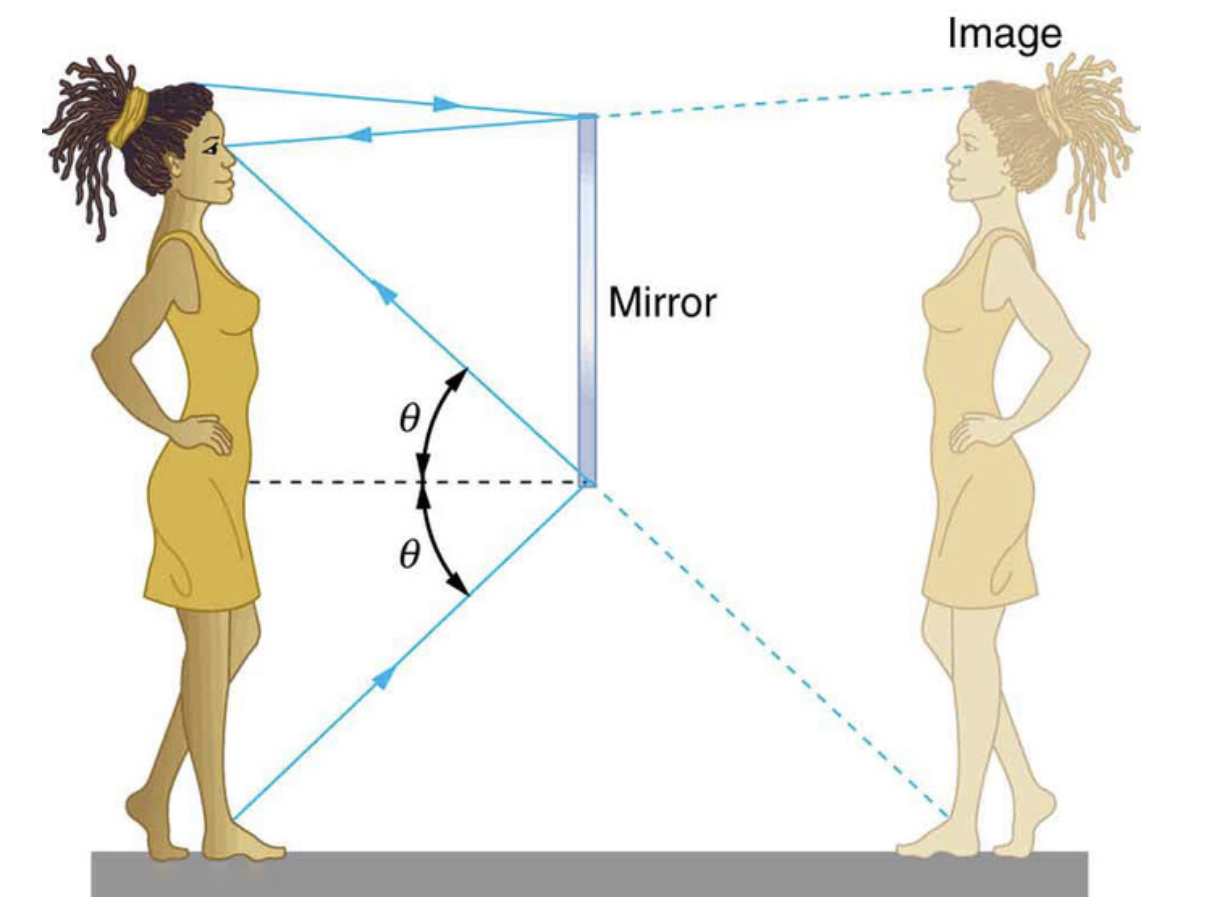The MCAT CARS Strategy Course begins January 28!

MCAT Content / Geometrical Optics / Reflection From Plane Surface Angle Of Incidence Equals Angle Of Reflection

### Reflection from plane surface: angle of incidence equals angle of reflection

Topic: Geometrical Optics

The law of reflection states that the angle of reflection equals the angle of incidence.

When light is bounced off of a material, such as a mirror, this is called a reflection. This is when a light ray, the incident ray, hits a reflective material and bounces off as the reflected ray at a specific angle. This is called the angle of reflection.The only way we can see an object that does not itself emit light is if that object reflects light. We usually notice reflections off a smooth surface.

However, light strikes different parts of a rough surface at different angles, and it is reflected in many different directions (“diffused”). Diffused light is what allows us to see a sheet of paper from any angle. Many objects, such as people, clothing, leaves, and walls, have rough surfaces and can be seen from all sides.

A mirror, on the other hand, has a smooth surface (compared with the wavelength of light) and reflects light at specific angles. Since the movement of the light rays can be shown geometrically, if a mirror is one-half your height, you could see your whole body in the reflection.The two rays shown are those that strike the mirror at just the correct angles to be reflected into the eyes of the viewer.The figure above helps illustrate how a flat mirror forms an image. Two rays are shown emerging from the same point, striking the mirror, and being reflected into the observer’s eye. The rays can diverge slightly, and both still get into the eye. If the rays are extrapolated backward, they seem to originate from a common point behind the mirror, locating the image. (The paths of the reflected rays into the eye are the same as if they had come directly from that point behind the mirror.) Using the law of reflection—the angle of reflection equals the angle of incidence—we can see that the image and object are the same distance from the mirror. This is a virtual image, since it cannot be projected—the rays only appear to originate from a common point behind the mirror. Obviously, if you walk behind the mirror, you cannot see the image, since the rays do not go there. But in front of the mirror, the rays behave exactly as if they had come from behind the mirror, so that is where the image is situated.

Practice Questions

A mirror in an operating room

An experiment with light rays and a prism

MCAT Official Prep (AAMC)

Physics Online Flashcards Question 19

Physics Question Pack Passage 10 Question 62

Key Points

• The angle of reflection equals the angle of incidence.

• Light strikes different parts of a rough surface at different angles and is reflected, or diffused, in many different directions.

• A mirror has a smooth surface (compared with the wavelength of light) and so reflects light at specific angles.

Key Terms

Reflection: the property of a propagated wave being thrown back from a surface.

Incident ray: light ray towards the surface

Reflected ray: light ray that bounced off the surface

Billing Information